Definitions
 Home | Definitions | Properties | Interpretations | Applications | Gambling | Software | Books

The classical definition of probability

Let us consider an urn U that contains n balls, from which m balls are white and n – m  balls are black. A ball is drawn at random. We have n elementary events. Let A be the event the drawn ball is white. This event can occurs in m tests,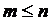.

Definition:  Call the probability of event A the ratio between the number of situations (tests) favorable for A to occur and the number of equally possible situations. Therefore,  P = m/n.

This is the classical, dictionary definition of probability. It can only be used in experiments having equally possible elementary events.

Probability on a finite field of events

In the case of an arbitrary finite field of events {Ω, Σ}, a probability on this field is defined as follows:

Definition:  Call probability on Σ a function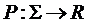that satisfies the following axioms:
(1)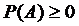, for any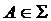;
(2)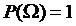;
(3), for anythat.

Axiom (3) can be generalized through recurrence to any finite number of mutually exclusive events: If,(i, j = 1, … , n), then.

Definition:  A finite field of events {Ω, Σ}, structured with a probability P, is called a finite probability field (finite probability space) and is denoted by {Ω, Σ, P}.

Probability as a measure

Definition:  Call a σ-field of events a field of events {Ω, Σ} that has the countable additivity property: any countable union of events from Σ is still an event from Σ (if, then).

The definition corresponds to the definition of tribe in measure theory.

Definition:  Let {Ω, Σ} be a σ-field of events. Call probability on {Ω, Σ} a numerical positive function P, defined on Σ, which meets the following conditions:
1)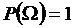2), for any countable family of mutually exclusive events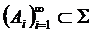.

A σ-field of events {Ω, Σ} along with a probability P is called a probability σ-field and is written {Ω, Σ, P}.

The two conditions from the definition of probability imply the axioms in the definition of measure. Therefore, the probability is a measure P with P(Ω) = 1, so it acquires all the properties of a measure.

Probability as a limit

There is an important theorem that illustrates the way in which probability models the hazard. We enunciate the Law of Large Numbers, not in its general mathematical form, in order to avoid having to define more complex concepts, but in an exemplified particular form, in a way that everyone can understand. The particular enunciation is the following classic result, known as Bernoulli’s Theorem:

Theorem: The relative frequency of the occurrence of a certain event in a sequence of independent experiments performed under identical conditions converges toward the probability of that event.

The theorem states that if A is an event,a sequence of independent experiments,the number of occurrences of event A after the first n experiments, then the sequence of non-negative numbers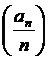is convergent and its limit is P(A):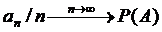The expressionis called frequency and the expressionis called relative frequency.

Example:
Consider the classical experiment of tossing a coin: Let A be the event the coin falls heads up. Obviously, P(A) = 1/2. Let us say that event A has the following occurrences:
– after the first throw, 0 occurrences, relative frequency 0/1;
– after the first two throws, 0 occurrences, relative frequency 0/2;
– after the first three throws, 1 occurrence, relative frequency 1/3;
– after the first four throws, 1 occurrence, relative frequency 1/4;
– after the first five throws, 2 occurrences, relative frequency 2/5;
……………………………………………………
after the first n throws,occurrences, frequency.

The Law of Large Numbers says that the sequence obtained at the right, namely 0, 0, 1/3, 1/4, 2/5, … is convergent toward 1/2. In other words, while n is growing, the relative frequency is approximating 1/2 with higher accuracy. The Law of Large Numbers confers on probability a property of limit.

Of course, the theorem does not provide information about the terms of the sequence of relative frequencies, but only about its limit. In other words, we cannot make a precise prediction on the event at a certain chronological moment, but we can only know the behavior of the relative frequency of occurrences at infinity.

You should retain:
●  Probability is nothing more than a measure; as length measures distance and area measures surface, probability measures aleatory events. As a measure, probability is in fact a function with certain properties, defined on the field of events generated by an experiment.
●  A probability is characterized not only by the specific function P, but by the entire aggregate the set of possible outcomes of the experiment – the field of generated events – function P, called probability field; probability makes no sense and cannot be calculated unless we initially rigorously define the probability field in which we operate. That is, we can say and compute the probability to be dealt certain cards at blackjack, but saying the probability of the sun not rising tomorrow makes no sense.
●  Probability is not a punctual numerical value; textually given an event, we cannot calculate its probability without including it in a more complex field of events. Probability as a number is in fact a limit, namely the limit of the sequence of relative frequencies of occurrences of the event to measure, within a sequence of independent experiments.

 Author The author of this page is Catalin Barboianu (PhD). Catalin is a games mathematician and problem gambling researcher, science writer and consultant for the mathematical aspects of gambling for the gaming industry and problem-gambling institutions. Profiles:   Linkedin   Google Scholar   Researchgate